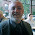One of the most amazing results of number theory

The prime numbers are not spaced evenly along the number line. What is the biggest gap between prime numbers?

There is no such "biggest gap." If we take the number, say, 3455324898588757997446990653578956897469994337854, we can always find a gap between prime numbers at least that large! (And I just typed a very large, completely random sequence of digits: this result holds for any number whatsoever!)

1.That doesn't strike me as amazing, at least in isolation - the prime numbers tend to get sparser as you go up, so it's not surprising that you can get arbitrarily large prime gaps. (Just as you get arbitrarily large gaps between, say, powers of 2.) But when you put that in context of other facts, like the fact that there seems to be infinitely twin primes, then you have something genuinely surprising.

In any case, what I would classify as one of the most remarkable results in number theory is Mihailascu's theorem, formerly known as the Catalan Conjecture: the only consecutive perfect powers are 8 and 9.

1."That doesn't strike me as amazing, at least in isolation..."

All right then, Mr. HardToImpress!

2.As Keshav says, this seems quite natural in view of say the Prime Number Theorem. Now the PNT itself, that's a doozy. (It seemed a doozy to Gauss :)) So I think what we have here is a difference in perspective. Keshav is a mathematician.

It can work the other way too. I can remember as an undergrad seeing the proof e^(i*pi) = -1. I was in a class with both pure math types like me and engineers. We mathies were (properly) amazed. That is an astounding result.

The engineers shrugged it off, wrote it down. (The prof gave a little speech about how this should impress you.)

3.What's more impressive is that if you swap the base and the power of one of those numbers, then you get the other: 2³ = 8 and 3² = 9.

Even more impressive than that is that every hyperoperation involving two twos will equal four: 2 + 2, 2 × 2, 2², and so on.